Courses

# State Variable Analysis - 2

## 10 Questions MCQ Test Topicwise Question Bank for Electrical Engineering | State Variable Analysis - 2

Description
This mock test of State Variable Analysis - 2 for Electrical Engineering (EE) helps you for every Electrical Engineering (EE) entrance exam. This contains 10 Multiple Choice Questions for Electrical Engineering (EE) State Variable Analysis - 2 (mcq) to study with solutions a complete question bank. The solved questions answers in this State Variable Analysis - 2 quiz give you a good mix of easy questions and tough questions. Electrical Engineering (EE) students definitely take this State Variable Analysis - 2 exercise for a better result in the exam. You can find other State Variable Analysis - 2 extra questions, long questions & short questions for Electrical Engineering (EE) on EduRev as well by searching above.
QUESTION: 1

### The state variable description of an autonomous system is, X = AX where X is a two-dimensional vector and A is a matrix given by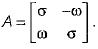The eigen values of A are

Solution:

Eigen values of A are given by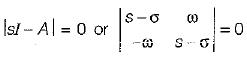or, (s - σ)2 + ω2 = 0
or,  s = σ ± jω
or,  s = σ + jω and s = σ - jω

QUESTION: 2

### The system equations are given by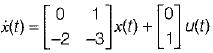y(t) = [1   0]x(t) The transfer function of the above system is

Solution:

Given,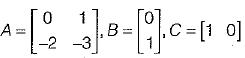Transfer function of the given system is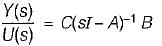Now,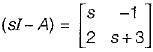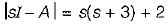= s2 + 3s + 2
Now,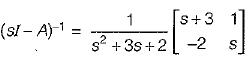Now,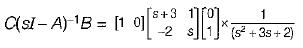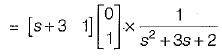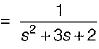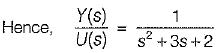QUESTION: 3

### Consider the following statements related to state space analysis of control systems: 1. The zeros of the system can be obtained from eigen value of the system matrix. 2. A system is said to be observable if every state x0 can be exactly determined from the measurement of the output ‘y’ over a finite interval of time 0 ≤ t ≤ tf. 3. The process by which transfer function changes to state diagram or state equations is called decomposition of the transfer function. 4. The state space techniques can be applied to linear and time invariant systems only. Which of the above statements are correct?

Solution:

The poles of the system can be obtained from eigen values of the system matrix. Hence, statement-1 is false.
State space techniques can be applied to linear or non-linear, time variant or time invariant systems.
Hence, statement-4 is false.

QUESTION: 4

Consider the system shown in figure below: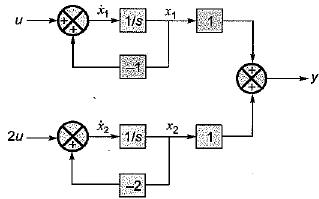The system is

Solution:

From given block diagram, the state equations can be written as:
x1 = -x1 + u and x2 = -2x2 + 2u
in matrix form,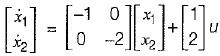Also, y = x1 + x2
In matrix form,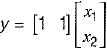Thus,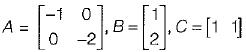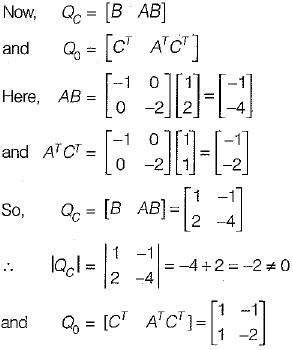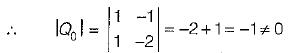Since, |Qc| ≠ 0 and |Q0| ≠ 0, therefore given system is both controllable and observable.

QUESTION: 5

Consider the following matrix: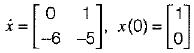x(t) is given by

Solution:

Given,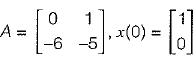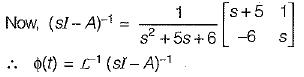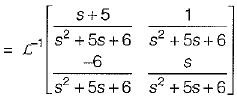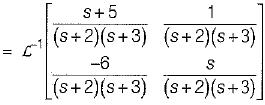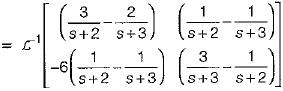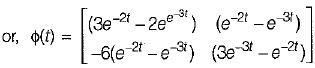So, x(t) = ϕ(t).x(0)
= state transition equation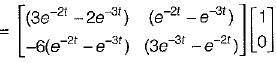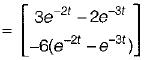QUESTION: 6

The transfer function of the system shown below is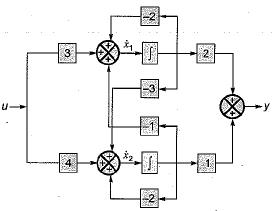Solution:

From given block diagram, the state equations can be written as: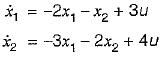Also, output equation is
y = 2x1 + x2
In matrix form, we have: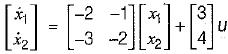and y = [2  1]x(t)
Now,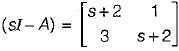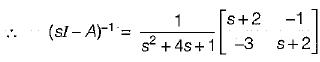∴ Transfer function,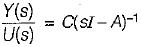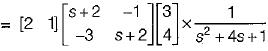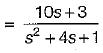QUESTION: 7

The state equation for the circuit shown below is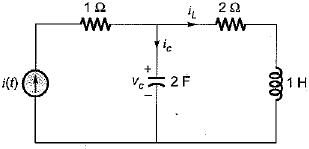Solution:

Let us select the state variables as Vc and iL.
Applying KVL in the mesh-2, we have: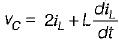or,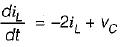.............(1)
Also, by applying KCL at the given node, we get: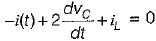or,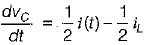..........(2)
From equations (1) and (2), state equations in matrix form can be written as: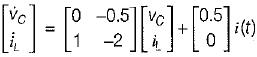QUESTION: 8

The state space representation of the system represented by the SFG shown below is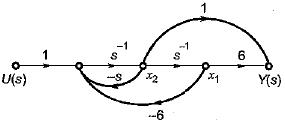Solution:

The state equations from the given signal flow graph can be written as: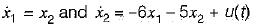In matrix form,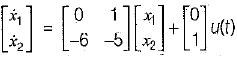Also, output is
y(t) = 6x1 + x2
In matrix form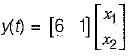QUESTION: 9

The state variable representation of a system is given by: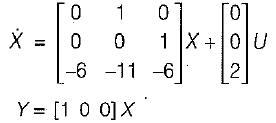The system is

Solution: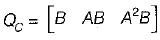Here,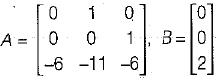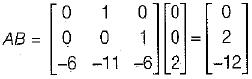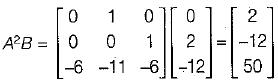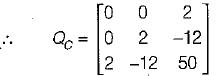So, IQCI = 0 - 0 + 2(0 - 4)
= - 8 ≠ 0
Hence, the system is controllable.
Also, Q0 = [CT ATCT (AT)2 CT]
Here,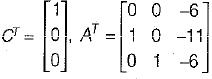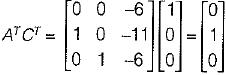and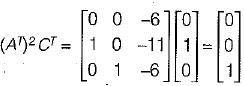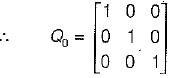So,= 1 ≠ 0
Since |Q0| ≠ 0, therefore given system is observable.

QUESTION: 10

The zeros of following system are located at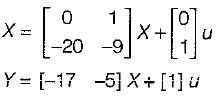Solution:

T.F.,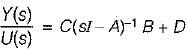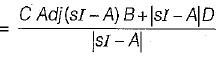Hence, zeros are at: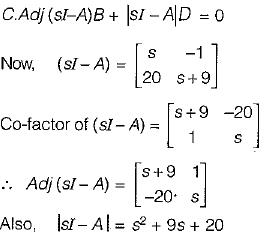So, zeros are at: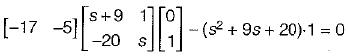or,  -17 -5s + s2 + 9s + 20 = 0
or,  s2 + 4s + 3 = 0
or,  (s+1) (s+3) = 0
or,  s = -1,- 3